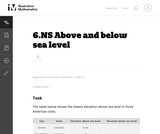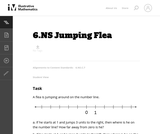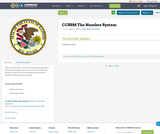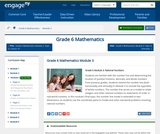# 12 Results

View
Selected filters:
• CCSS.Math.Content.6.NS.C.7Conditions of Use:
Remix and Share
Rating

Rational Numbers

Type of Unit: Concept

Prior Knowledge

Students should be able to:

Solve problems with positive rational numbers.
Plot positive rational numbers on a number line.
Understand the equal sign.
Use the greater than and less than symbols with positive numbers (not variables) and understand their relative positions on a number line.
Recognize the first quadrant of the coordinate plane.

Lesson Flow

The first part of this unit builds on the prerequisite skills needed to develop the concept of negative numbers, the opposites of numbers, and absolute value. The unit starts with a real-world application that uses negative numbers so that students understand the need for them. The unit then introduces the idea of the opposite of a number and its absolute value and compares the difference in the definitions. The number line and positions of numbers on the number line is at the heart of the unit, including comparing positions with less than or greater than symbols.

The second part of the unit deals with the coordinate plane and extends student knowledge to all four quadrants. Students graph geometric figures on the coordinate plane and do initial calculations of distances that are a straight line. Students conclude the unit by investigating the reflections of figures across the x- and y-axes on the coordinate plane.

Subject:
Mathematics
Numbers and Operations
Material Type:
Unit of Study
Provider:
PearsonConditions of Use:
Remix and Share
Rating

Subject:
Mathematics
Material Type:
Full Course
Provider:
Pearson
10/06/2016Conditions of Use:
Remix and Share
Rating

This is an activity with absolute value of integers. This is a fun card game that gets your kids involved with finding and comparing absolute value.

Subject:
Mathematics
Material Type:
Activity/Lab
Author:
Amie Chicko
01/28/2016Conditions of Use:
Remix and Share
Rating

Students identify whether an inequality statement is true or false using a number line to support their reasoning.Key ConceptsThe meaning of mThe meaning of n > m is that n is located to the right of m on a number line. The inequality statement n > m is read “n is greater than m.”To decide on the order of two numbers m and n, locate the numbers on a number line. If m is to the left of n, then m < n. If m is to the right of n, then m > n.Goals and Learning ObjectivesState whether an inequality is true or false.Use a number line to prove that an inequality is true or false.

Subject:
Numbers and Operations
Material Type:
Lesson Plan
Provider:
Pearson
09/21/2015Rating

Navigate the number line while diving amidst shipwrecks and sunken ruins. Will you find a pearl, or an old boot? Watch out for the electric eel! Pearl Diver teaches properties of numbers, how to plot numbers, how to visualize quantity on the number line, how to order numbers, and how to use the number line as a visual model for mathematical operations.

Subject:
Mathematics
Material Type:
Game
Interactive
Provider:
Learning Games Lab
Author:
NMSU Learning Games Lab
07/20/2015Conditions of Use:
No Strings Attached
Rating

The purpose of this task is to help students interpret signed numbers in a context as a magnitude and a direction and to make sense of the absolute value of a signed number as its magnitude.

Subject:
Mathematics
Material Type:
Activity/Lab
Provider:
Illustrative Mathematics
Provider Set:
Illustrative Mathematics
Author:
Illustrative Mathematics
05/01/2012Conditions of Use:
No Strings Attached
Rating

This purpose of this task is to help students understand the absolute value of a number as its distance from 0 on the number line. The context is not realistic, nor is meant to be; it is a thought experiment to help students focus on the relative position of numbers on the number line.

Subject:
Mathematics
Material Type:
Activity/Lab
Provider:
Illustrative Mathematics
Provider Set:
Illustrative Mathematics
Author:
Illustrative Mathematics
05/01/2012
RemixConditions of Use:
Remix and Share
Rating

This resource links to both the Fractions progression document published by the Common Core Writing Teams in June 2011 and the Module posted on the Illustrative Mathematics website.

Material Type:
Assessment
Homework/Assignment
Teaching/Learning Strategy
Author:
ISBE Math Specialists
08/08/2019Conditions of Use:
Remix and Share
Rating

Students analyze whether given statements are possible or impossible using their definitions of absolute value and the opposite of a number. If the statements are possible, students give an example of a pair of numbers that fit the statement. If the statements are impossible, students explain why.Key ConceptsA number and the opposite of the number always have the same absolute value.In general, taking the opposite of n changes the sign of n. For example, the opposite of 3 is −3.In general, taking the absolute value of n gives a number |n|, which is always positive. For example, |3| = 3 and |−3| = 3.Since the opposite of 0 is 0 (which is neither positive nor negative), therefore −0 = 0. The number 0 is the only number which is its own opposite.Goals and Learning ObjectivesFind pairs of numbers that satisfy different statements about absolute values and/or the opposites of numbers.State when it is impossible to find a pair of numbers that satisfies the statement and explain why.

Subject:
Numbers and Operations
Material Type:
Lesson Plan
Provider:
Pearson
09/21/2015Conditions of Use:
Remix and Share
Rating

Students are familiar with the number line and determining the location of positive fractions, decimals, and whole numbers from previous grades. Students extend the number line (both horizontally and vertically) in Module 3 to include the opposites of whole numbers. The number line serves as a model to relate integers and other rational numbers to statements of order in real-world contexts. In this module's final topic, the number line model is extended to two-dimensions, as students use the coordinate plane to model and solve real-world problems involving rational numbers.

Subject:
Numbers and Operations
Material Type:
Module
Provider:
New York State Education Department
Provider Set:
EngageNY
10/20/2013Conditions of Use:
Remix and Share
Rating

Students revise their work on the Self Check based on feedback from the teacher and their peers.Key ConceptsConcepts from previous lessons are integrated into this assessment task: integers, absolute value, and comparing numbers. Students apply their knowledge, review their work, and make revisions based on feedback from the teacher and their peers. This process creates a deeper understanding of the concepts.Goals and Learning ObjectivesApply your knowledge of integers, absolute value, and comparing numbers to solve problems.Track and review your choice of strategy when problem solving.

Subject:
Numbers and Operations
Material Type:
Lesson Plan
Provider:
Pearson
09/21/2015Conditions of Use:
Remix and Share
Rating

Students answer questions about low temperatures recorded in Barrow, Alaska, to understand when to use negative numbers and when to use the absolute values of numbers.Key ConceptsThe absolute value of a number is its distance from 0 on a number line.The absolute value of a number n is written |n| and is read as “the absolute value of n.”A number and the opposite of the number always have the same absolute value. As shown in the diagram, |3| = 3 and |−3| = 3.In general, taking the opposite of n changes the sign of n. For example, the opposite of 3 is –3.In general, taking the absolute value of n gives a number, |n|, that is always positive unless n = 0. For example, |3| = 3 and |−3| = 3.The absolute value of 0 is 0, which is neither positive nor negative: |0| = 0.Goals and Learning ObjectivesUnderstand when to talk about a number as negative and when to talk about the absolute value of a number.Locate the absolute value of a and the absolute value of b on a number line that shows the location of a and b in different places in relation to 0.

Subject:
Numbers and Operations
Material Type:
Lesson Plan
Provider:
Pearson